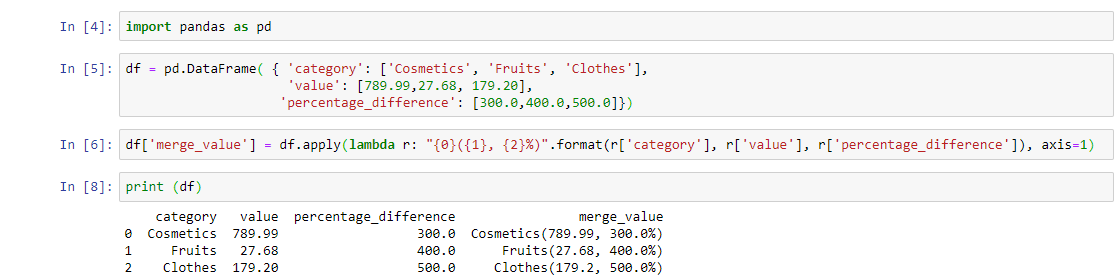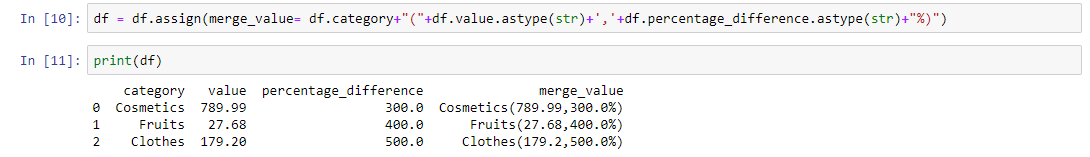2 views
in Python

I have a dataframe.

category       value        percentage_difference

Cosmetics    789.99      300.0

Fruits           27.68         400.0

Clothes       179.20       500.0

I want to add fourth column so that its value is category(value, percentage)

category      value          percentage_difference      merge_value

Cosmetics   789.99       300.0                                   Cosmetic(789.99, 300%)

Fruits          27.68          400.0                                  Fruits(27.68, 400%)

Clothes      179.20        500.0                                  Clothes(179.20, 500%

I can do this using for loop, is there any other efficient method that can perform the task without using for loop?

by (106k points)
edited by

There are few ways to do so like:

The first approach that we can perform is too use pandas dataframe that will do this task as follows:-

df = pd.DataFrame( { 'category': ['Cosmetics', 'Fruits', 'Clothes'], 'value': [789.99,27.68, 179.20], 'percentage_difference': [300.0,400.0,500.0]})

df['merge_value'] = df.apply(lambda r: "{0}({1}, {2}%)".format(r['category'], r['value'], r['percentage_difference']), axis=1)Other approach  to do this problem is just add the column as strings:

df=df.assign(merge_value= df.category+"("+df.value.astype(str)+','+df.percentage_difference.astype(str)+"%)")

print(df)